# Chapter 4 Digital Transmission Digital Transmission q The

• Slides: 74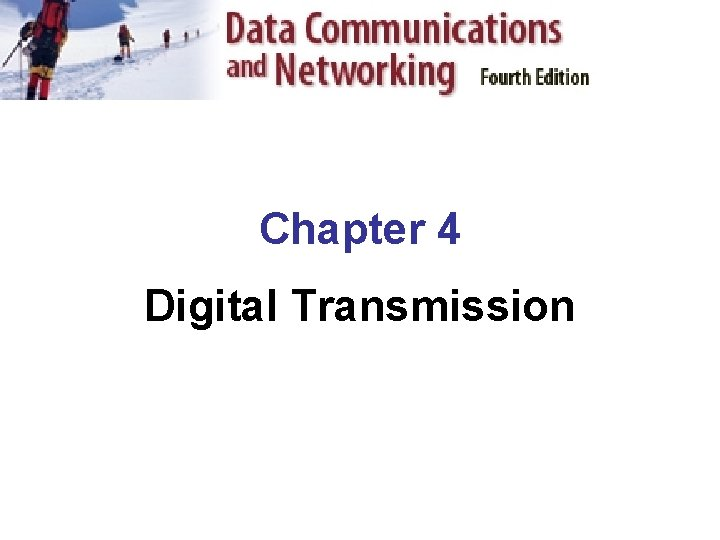Chapter 4 Digital Transmission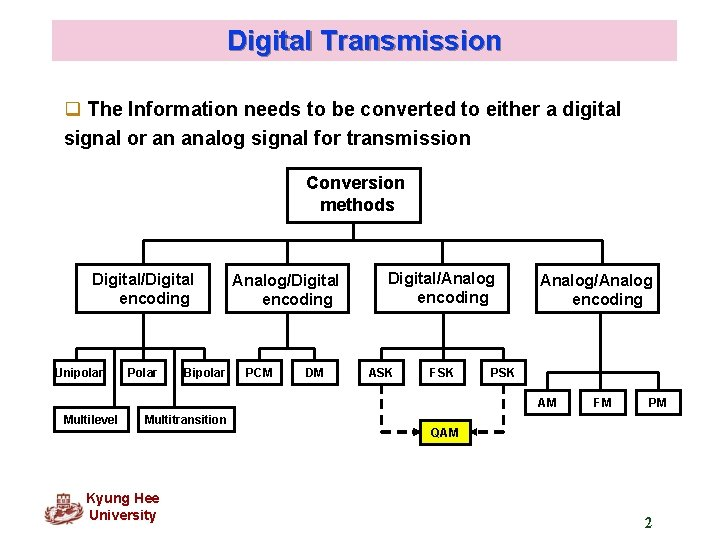Digital Transmission q The Information needs to be converted to either a digital signal or an analog signal for transmission Conversion methods Digital/Digital encoding Unipolar Polar Bipolar Analog/Digital encoding PCM DM Digital/Analog encoding ASK FSK Analog/Analog encoding PSK AM Multilevel Multitransition Kyung Hee University FM PM QAM 2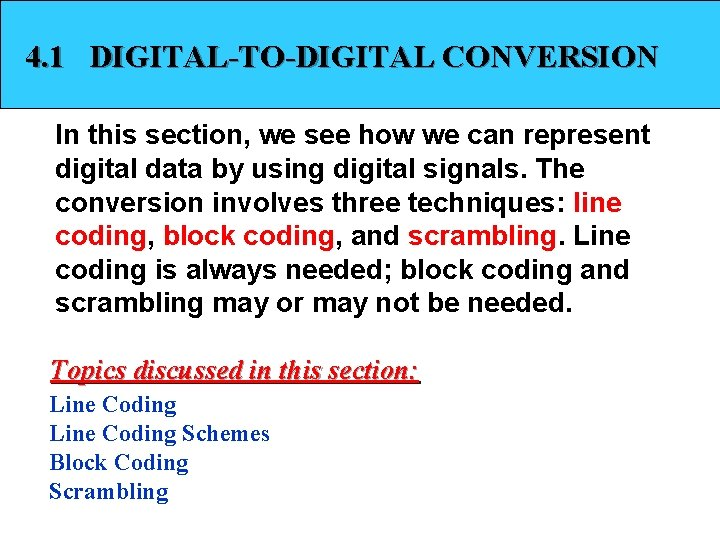4. 1 DIGITAL-TO-DIGITAL CONVERSION In this section, we see how we can represent digital data by using digital signals. The conversion involves three techniques: line coding, block coding, and scrambling. Line coding is always needed; block coding and scrambling may or may not be needed. Topics discussed in this section: Line Coding Schemes Block Coding ScramblingDigital-to-Digital Conversion q Converts sequence of bits to a digital signal Figure 4. 1 Line coding and decoding Kyung Hee University 4Characteristics of Line Coding q Signal element vs. data element q Data rate vs. bit rate q dc components q Self-synchronization Kyung Hee University 5Data element versus Signal element q Data element – the smallest entity that can represent a piece of information : this is the bit. q Signal element – carries data element. A signal element is the shortest unit of a digital signal. q. Data elements are being carried; signal elements are the carriers. Kyung Hee University 6Data element versus Signal element Figure 4. 2 Signal element versus data element Kyung Hee University 7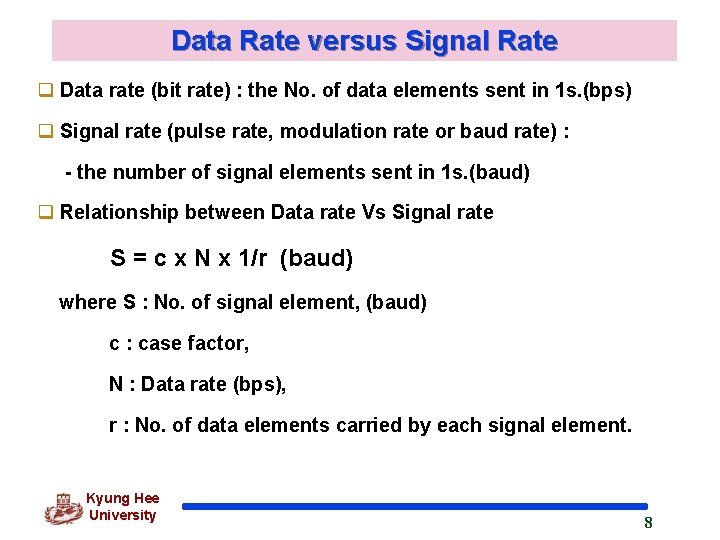Data Rate versus Signal Rate q Data rate (bit rate) : the No. of data elements sent in 1 s. (bps) q Signal rate (pulse rate, modulation rate or baud rate) : - the number of signal elements sent in 1 s. (baud) q Relationship between Data rate Vs Signal rate S = c x N x 1/r (baud) where S : No. of signal element, (baud) c : case factor, N : Data rate (bps), r : No. of data elements carried by each signal element. Kyung Hee University 8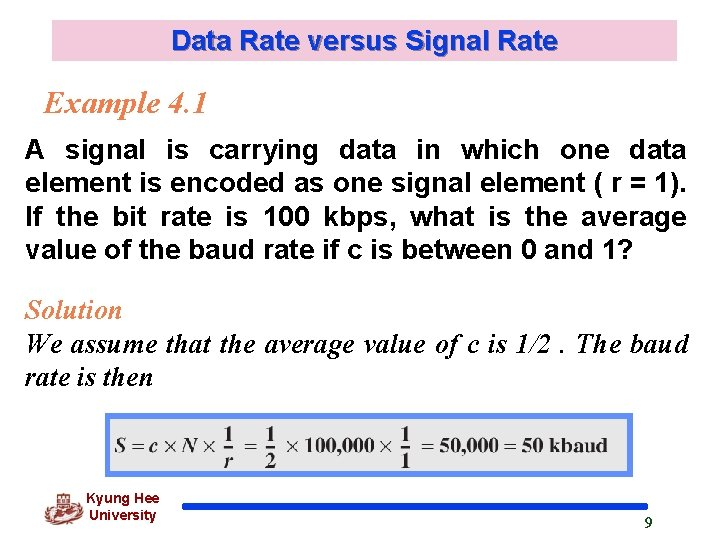Data Rate versus Signal Rate Example 4. 1 A signal is carrying data in which one data element is encoded as one signal element ( r = 1). If the bit rate is 100 kbps, what is the average value of the baud rate if c is between 0 and 1? Solution We assume that the average value of c is 1/2. The baud rate is then Kyung Hee University 9Bandwidth Note Although the actual bandwidth of a digital signal is infinite, the effective bandwidth is finite. * A digital signal that carries information is nonperiodic. So, bandwidth of nonperiodic signal is continuous with an infinite range theoretically. Kyung Hee University 10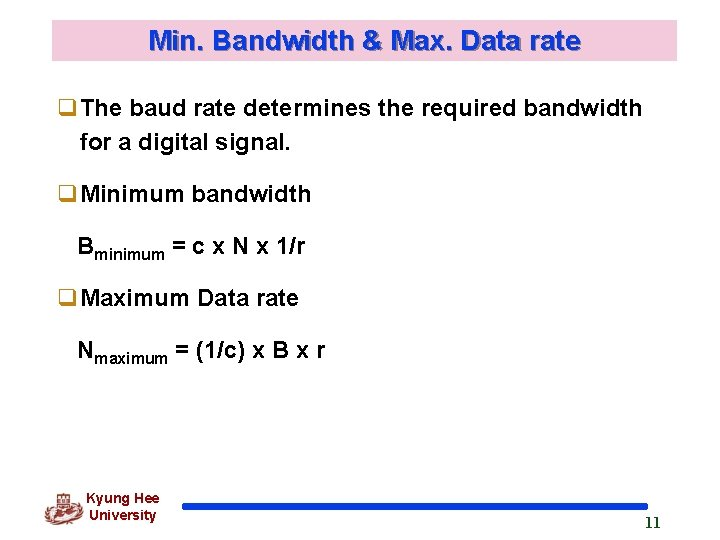Min. Bandwidth & Max. Data rate q. The baud rate determines the required bandwidth for a digital signal. q. Minimum bandwidth Bminimum = c x N x 1/r q. Maximum Data rate Nmaximum = (1/c) x B x r Kyung Hee University 11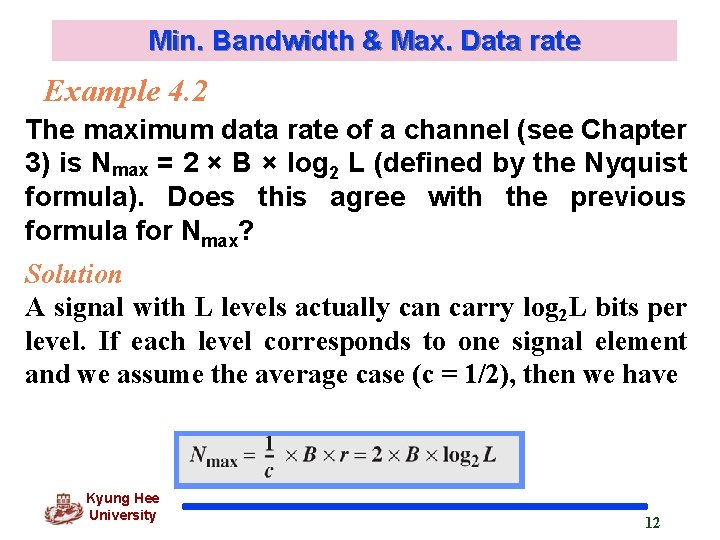Min. Bandwidth & Max. Data rate Example 4. 2 The maximum data rate of a channel (see Chapter 3) is Nmax = 2 × B × log 2 L (defined by the Nyquist formula). Does this agree with the previous formula for Nmax? Solution A signal with L levels actually can carry log 2 L bits per level. If each level corresponds to one signal element and we assume the average case (c = 1/2), then we have Kyung Hee University 12DC Components q When the voltage level in a digital signal is constant for a while, the spectrum creates very low frequencies. These frequencies are around zero, called DC(direct current) components. q Some systems (such as transformer) will not allow passage of DC component q A telephone line cannot pass frequencies below 200 Hz Kyung Hee University 13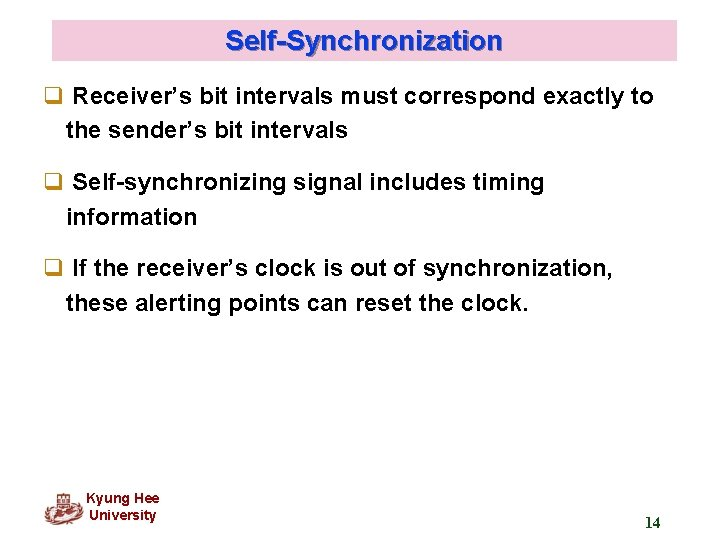Self-Synchronization q Receiver’s bit intervals must correspond exactly to the sender’s bit intervals q Self-synchronizing signal includes timing information q If the receiver’s clock is out of synchronization, these alerting points can reset the clock. Kyung Hee University 14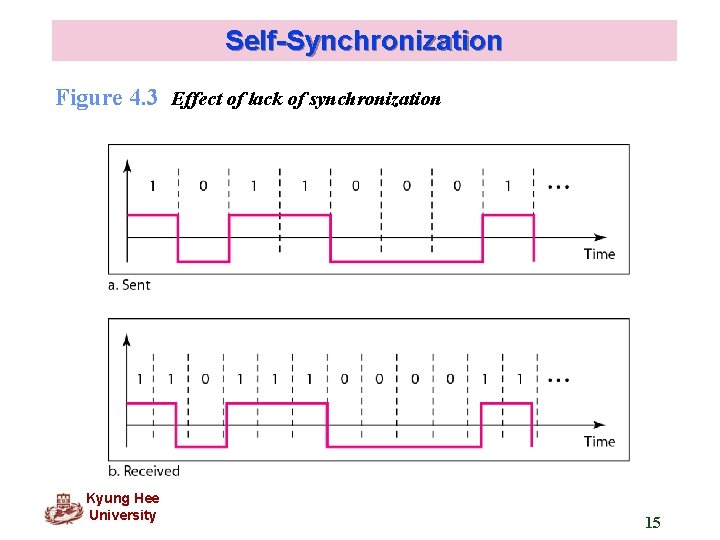Self-Synchronization Figure 4. 3 Effect of lack of synchronization Kyung Hee University 15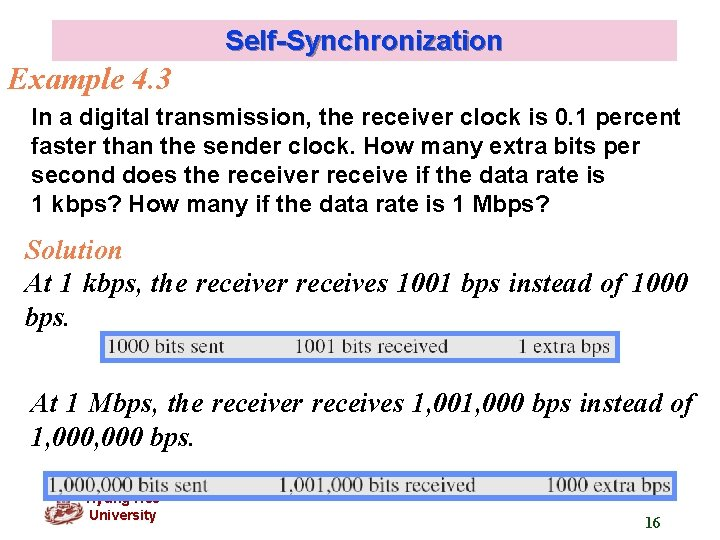Self-Synchronization Example 4. 3 In a digital transmission, the receiver clock is 0. 1 percent faster than the sender clock. How many extra bits per second does the receiver receive if the data rate is 1 kbps? How many if the data rate is 1 Mbps? Solution At 1 kbps, the receiver receives 1001 bps instead of 1000 bps. At 1 Mbps, the receiver receives 1, 000 bps instead of 1, 000 bps. Kyung Hee University 16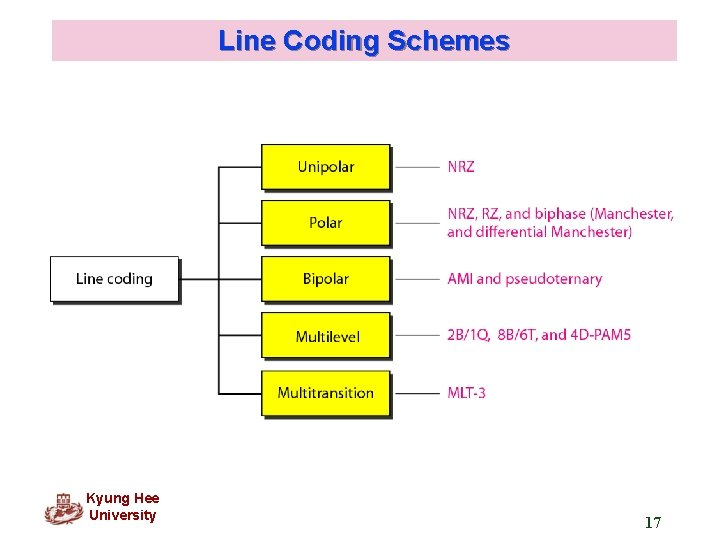Line Coding Schemes Kyung Hee University 17Line Coding Schemes Figure 4. 5 Unipolar NRZ scheme q Unipolar encoding uses only one voltage level. Kyung Hee University 18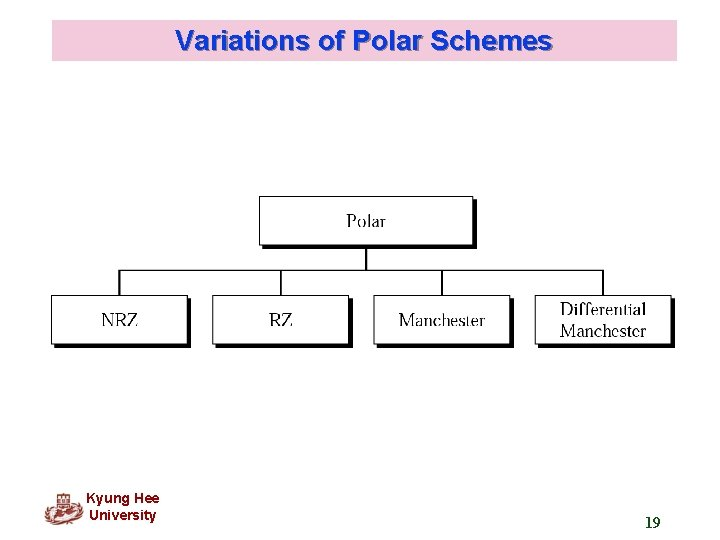Variations of Polar Schemes Kyung Hee University 19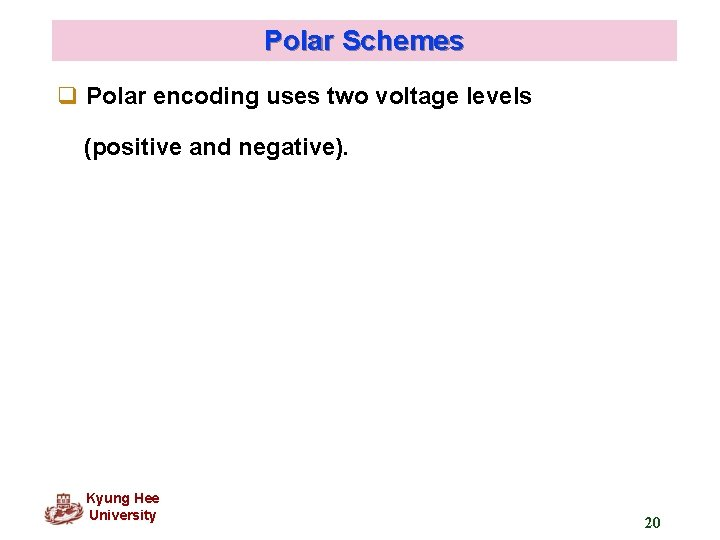Polar Schemes q Polar encoding uses two voltage levels (positive and negative). Kyung Hee University 20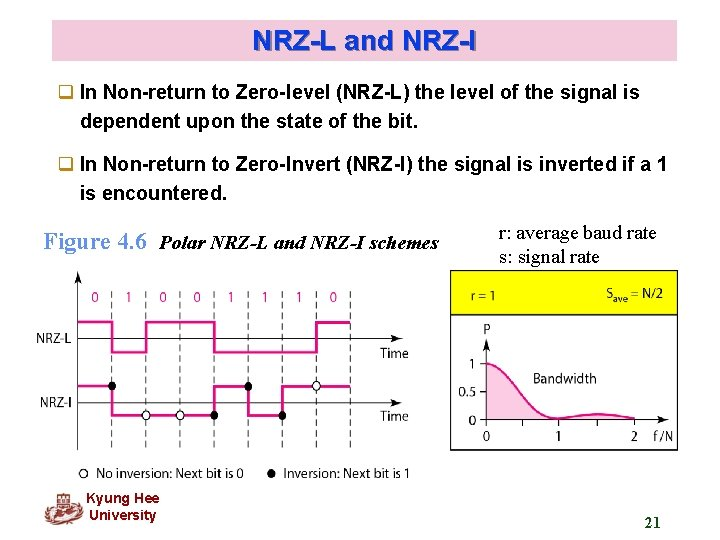NRZ-L and NRZ-I q In Non-return to Zero-level (NRZ-L) the level of the signal is dependent upon the state of the bit. q In Non-return to Zero-Invert (NRZ-I) the signal is inverted if a 1 is encountered. Figure 4. 6 Polar NRZ-L and NRZ-I schemes Kyung Hee University r: average baud rate s: signal rate 21Note In NRZ-L the level of the voltage determines the value of the bit. In NRZ-I the inversion or the lack of inversion determines the value of the bit.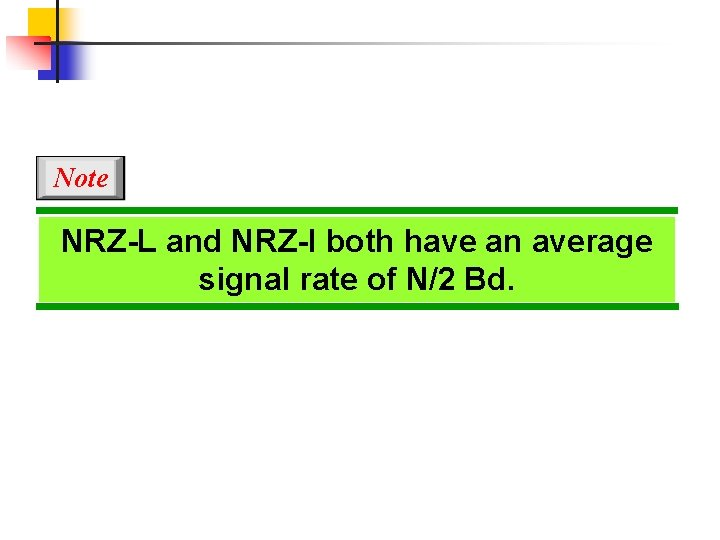Note NRZ-L and NRZ-I both have an average signal rate of N/2 Bd.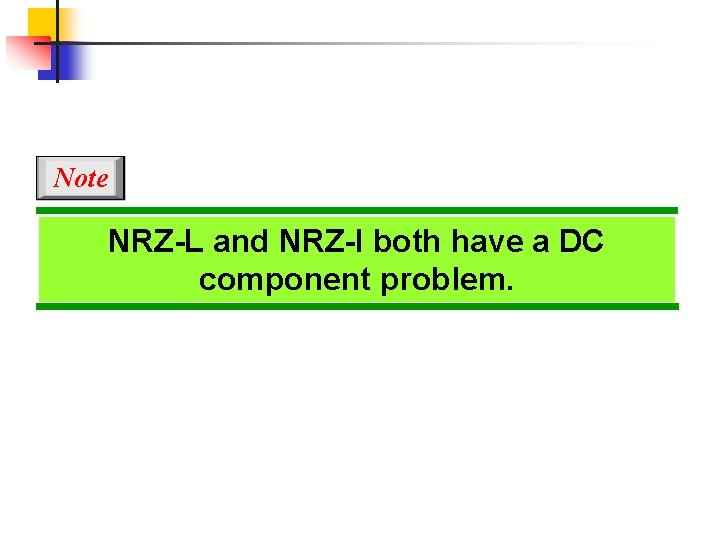Note NRZ-L and NRZ-I both have a DC component problem.Example 4. 4 A system is using NRZ-I to transfer 1 Mbps data. What are the average signal rate and minimum bandwidth? Solution The average signal rate is S = N/2 = 500 kbaud. The minimum bandwidth for this average baud rate is Bmin = S = 500 k. Hz.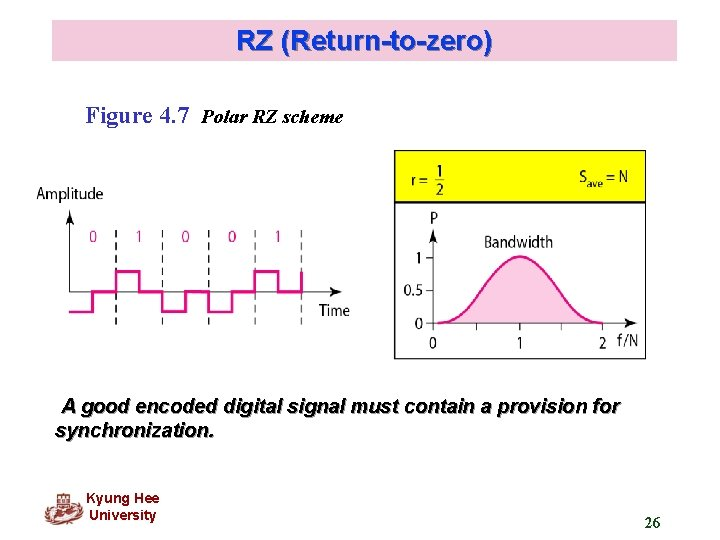RZ (Return-to-zero) Figure 4. 7 Polar RZ scheme A good encoded digital signal must contain a provision for synchronization. Kyung Hee University 26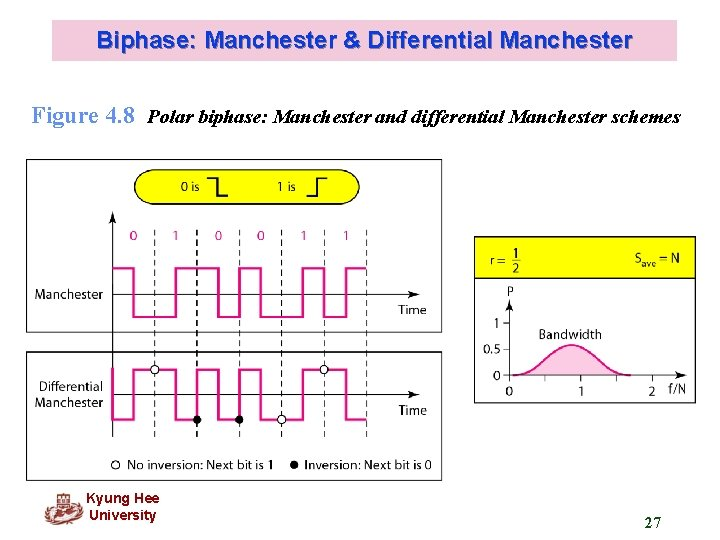Biphase: Manchester & Differential Manchester Figure 4. 8 Polar biphase: Manchester and differential Manchester schemes Kyung Hee University 27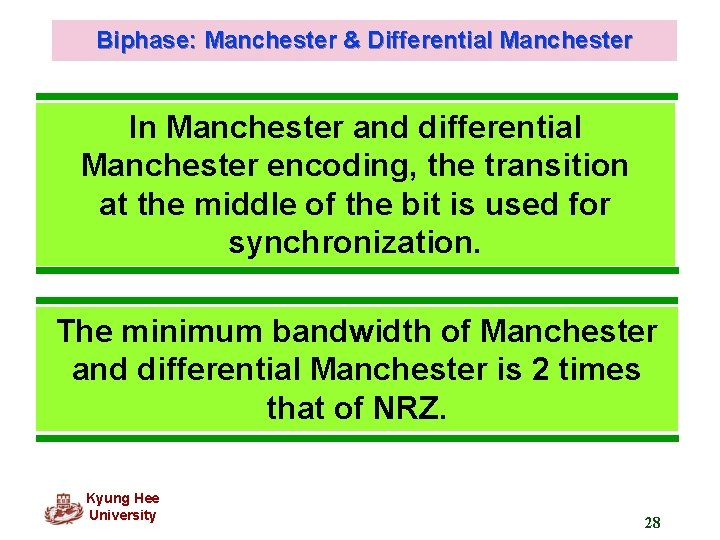Biphase: Manchester & Differential Manchester In Manchester and differential Manchester encoding, the transition at the middle of the bit is used for synchronization. The minimum bandwidth of Manchester and differential Manchester is 2 times that of NRZ. Kyung Hee University 28Bipolar Schemes q In bipolar encoding, we use three levels: - positive, zero, and negative. q Bipolar schemes: AMI and pseudoternary Kyung Hee University 29Multilevel Schemes q The desire to increase the data speed or decrease the required bandwidth has resulted in the creation of many scheme. q The goal is to increase the number of bits per baud by encoding a pattern of m data elements into a pattern of n signal elements. In m. Bn. L schemes, a pattern of m data elements is encoded as a pattern of n signal elements in which 2 m ≤ Ln. Kyung Hee University 30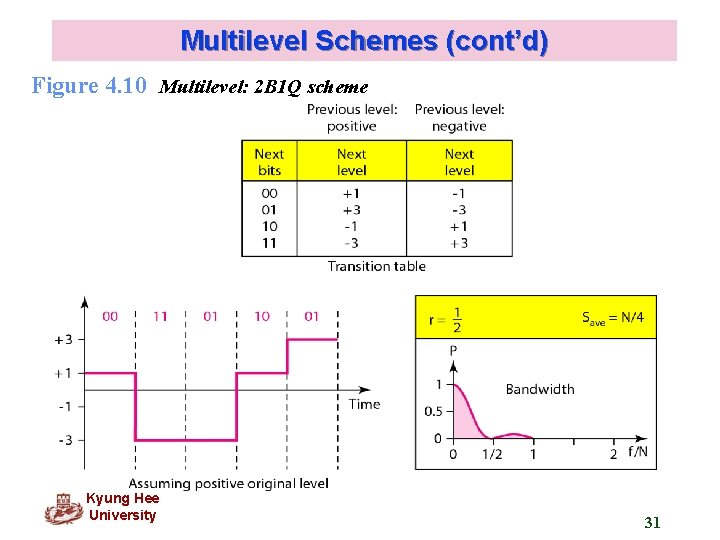Multilevel Schemes (cont’d) Figure 4. 10 Multilevel: 2 B 1 Q scheme Kyung Hee University 31Multilevel Schemes (cont’d) Figure 4. 11 Multilevel: 8 B 6 T scheme • 8 bit code = 28 = 256 • 6 bit ternary = 36 = 729 Kyung Hee University 32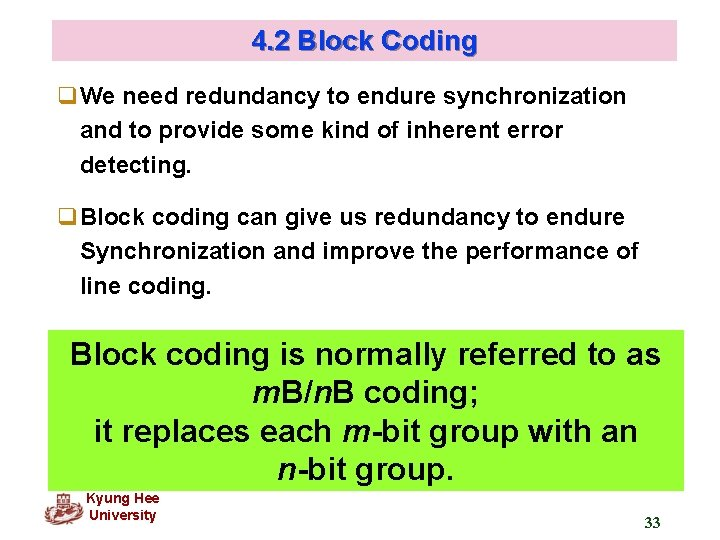4. 2 Block Coding q. We need redundancy to endure synchronization and to provide some kind of inherent error detecting. q. Block coding can give us redundancy to endure Synchronization and improve the performance of line coding. Block coding is normally referred to as m. B/n. B coding; it replaces each m-bit group with an n-bit group. Kyung Hee University 33Block Coding (cont’d) q Steps in transmission Step 1: Division l divide sequence of bits into groups of m bits Step 2: Substitution l substitute an m-bit code for an n-bit group Step 3: Line Coding l create the signal Kyung Hee University 34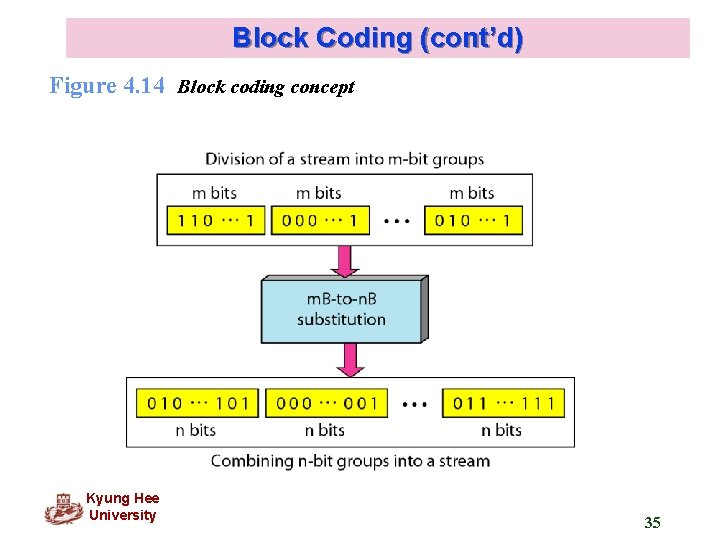Block Coding (cont’d) Figure 4. 14 Block coding concept Kyung Hee University 35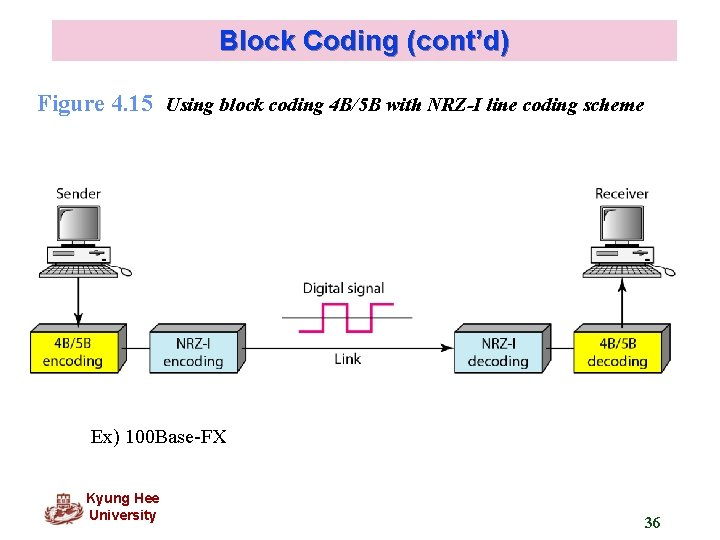Block Coding (cont’d) Figure 4. 15 Using block coding 4 B/5 B with NRZ-I line coding scheme Ex) 100 Base-FX Kyung Hee University 36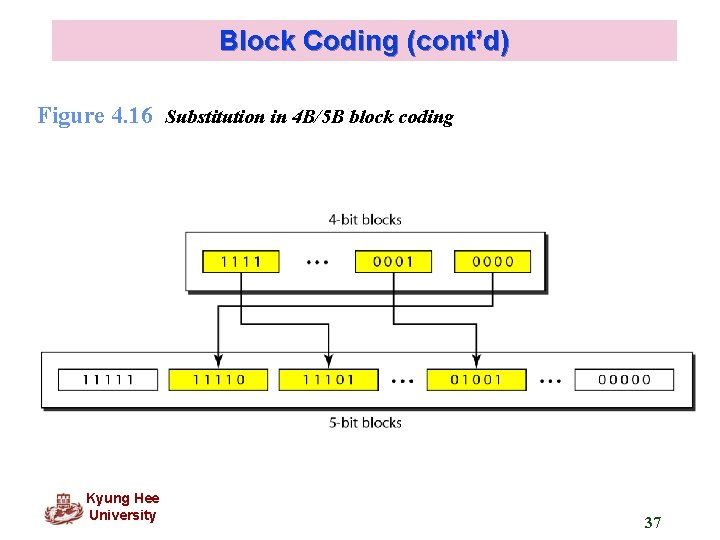Block Coding (cont’d) Figure 4. 16 Substitution in 4 B/5 B block coding Kyung Hee University 37Block Coding (cont’d) Table 4. 2 4 B/5 B mapping codes Kyung Hee University 38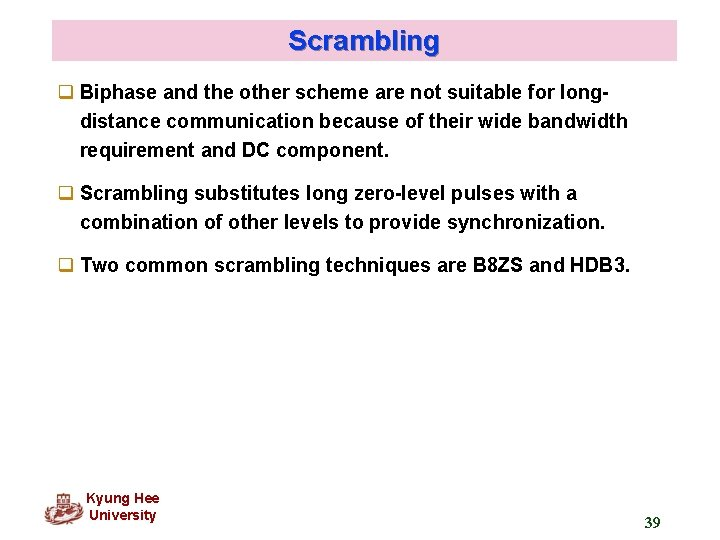Scrambling q Biphase and the other scheme are not suitable for longdistance communication because of their wide bandwidth requirement and DC component. q Scrambling substitutes long zero-level pulses with a combination of other levels to provide synchronization. q Two common scrambling techniques are B 8 ZS and HDB 3. Kyung Hee University 39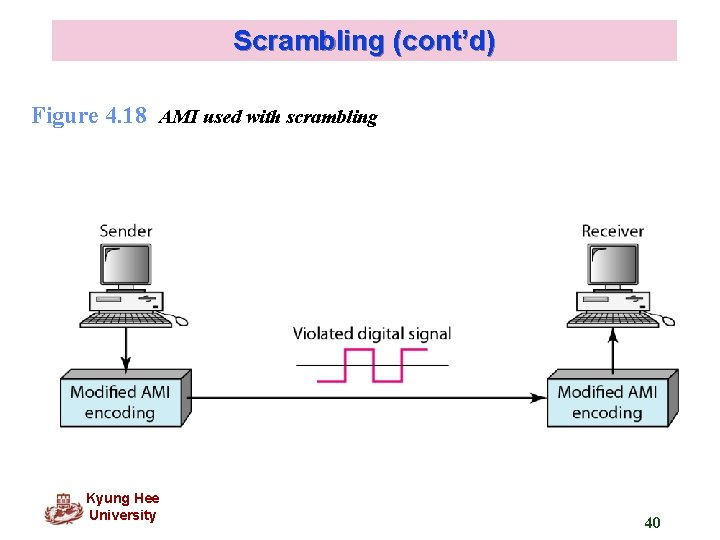Scrambling (cont’d) Figure 4. 18 AMI used with scrambling Kyung Hee University 40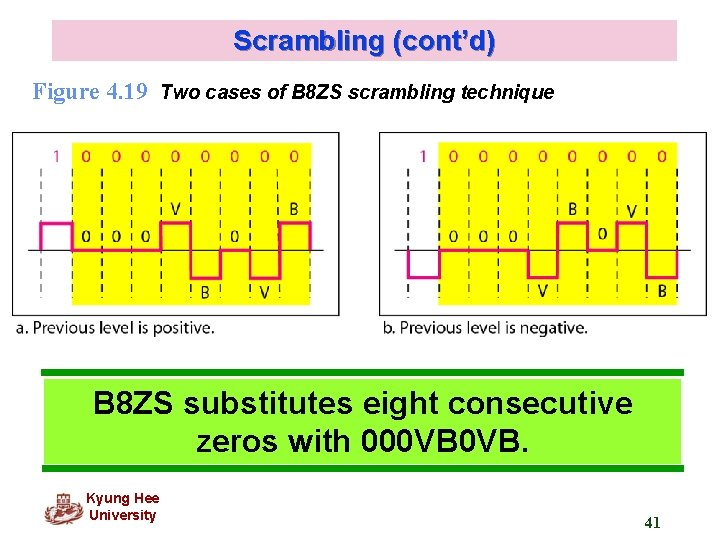Scrambling (cont’d) Figure 4. 19 Two cases of B 8 ZS scrambling technique B 8 ZS substitutes eight consecutive zeros with 000 VB 0 VB. Kyung Hee University 41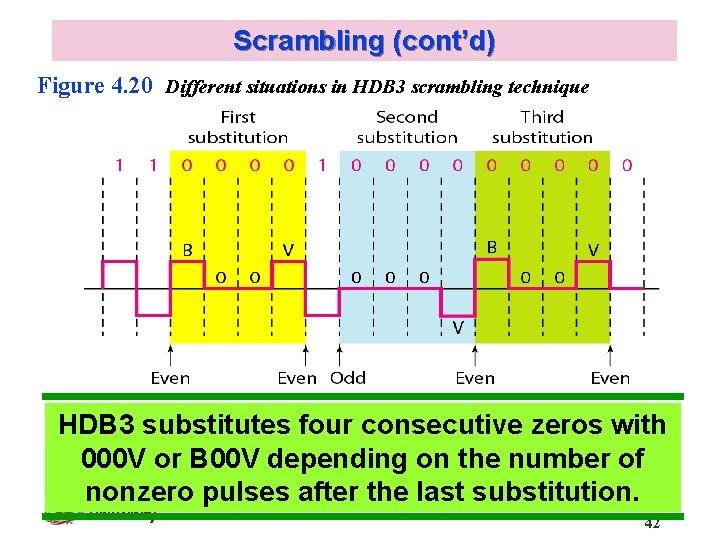Scrambling (cont’d) Figure 4. 20 Different situations in HDB 3 scrambling technique HDB 3 substitutes four consecutive zeros with 000 V or B 00 V depending on the number of nonzero pulses after the last substitution. Kyung Hee University 424. 2 ANALOG-TO-DIGITAL CONVERSION We have seen in Chapter 3 that a digital signal is superior to an analog signal. The tendency today is to change an analog signal to digital data. In this section we describe two techniques, pulse code modulation and delta modulation. Topics discussed in this section: Pulse Code Modulation (PCM) Delta Modulation (DM)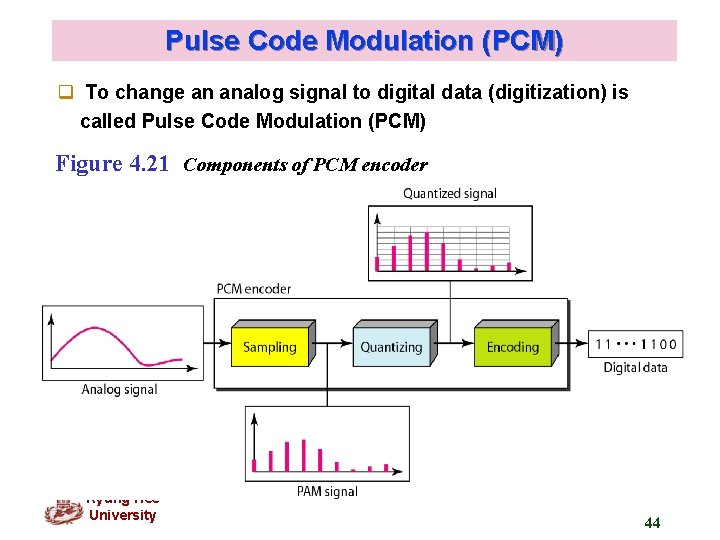Pulse Code Modulation (PCM) q To change an analog signal to digital data (digitization) is called Pulse Code Modulation (PCM) Figure 4. 21 Components of PCM encoder Kyung Hee University 44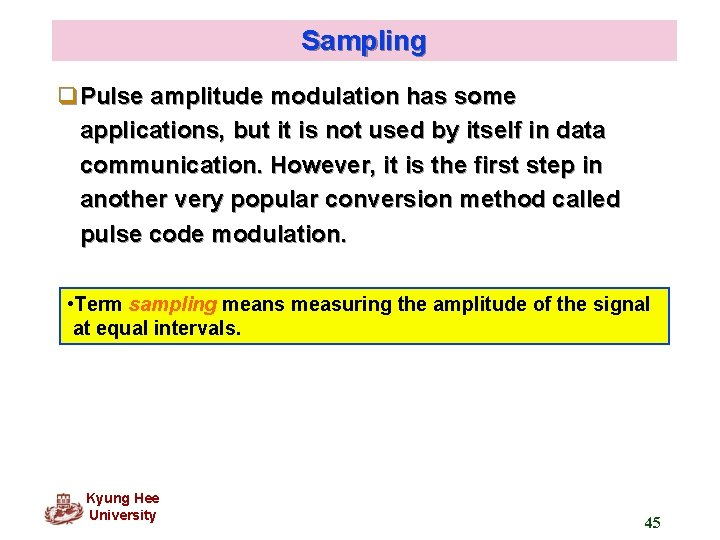Sampling q. Pulse amplitude modulation has some applications, but it is not used by itself in data communication. However, it is the first step in another very popular conversion method called pulse code modulation. • Term sampling means measuring the amplitude of the signal at equal intervals. Kyung Hee University 45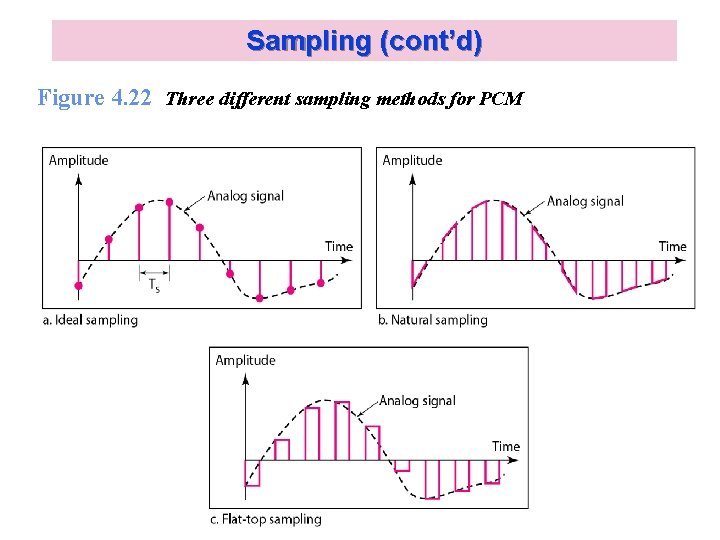Sampling (cont’d) Figure 4. 22 Three different sampling methods for PCM Kyung Hee University 46Sampling (cont’d) Note According to the Nyquist theorem, the sampling rate must be at least 2 times the highest frequency contained in the signal. Kyung Hee University 47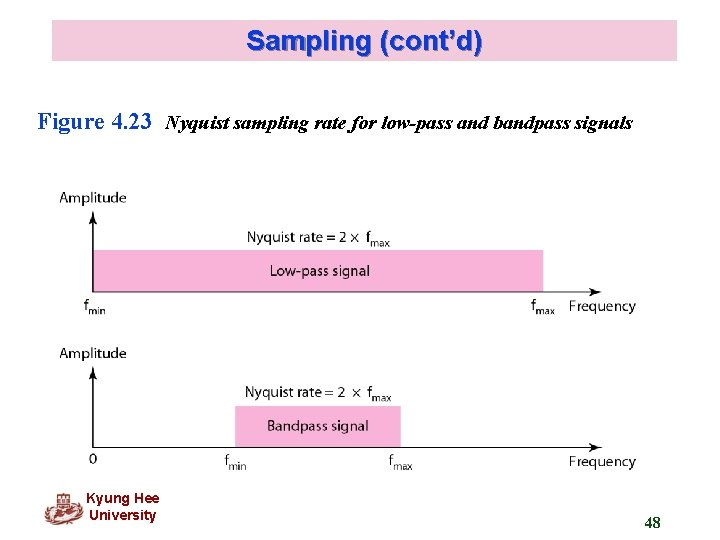Sampling (cont’d) Figure 4. 23 Nyquist sampling rate for low-pass and bandpass signals Kyung Hee University 48Sampling (cont’d) Example 4. 6 For an intuitive example of the Nyquist theorem, let us sample a simple sine wave at three sampling rates: fs = 4 f (2 times the Nyquist rate), fs = 2 f (Nyquist rate), and fs = f (one-half the Nyquist rate). Figure 4. 24 shows the sampling and the subsequent recovery of the signal. It can be seen that sampling at the Nyquist rate can create a good approximation of the original sine wave (part a). Oversampling in part b can also create the same approximation, but it is redundant and unnecessary. Sampling below the Nyquist rate (part c) does not produce a signal that looks like the original sine wave.Sampling (cont’d) Figure 4. 24 Recovery of a sampled sine wave for different sampling rates Kyung Hee University 50Sampling (cont’d) Example 4. 9 Telephone companies digitize voice by assuming a maximum frequency of 4000 Hz. The sampling rate therefore is 8000 samples per second. Kyung Hee University 51Quantization q The result of sampling is a series of pulses with amplitude values between the max. and min. amplitudes of the signal. q These values cannot be used in the encoding process. q The following are the steps in quantization: Assume the original analog signal has instantaneous amplitudes between Vmin and Vmax. Divide the range into L zones, each of height Δ (delta) Δ = (Vmax - Vmin) / L Assign quantized values of 0 to L-1 to the midpoint of each zone Approximate the value of the sample amplitude to the quantized values. q Quantization Error SNRd. B = 6. 02 nb + 1. 76 d. B Kyung Hee University Nb : bits per sample 52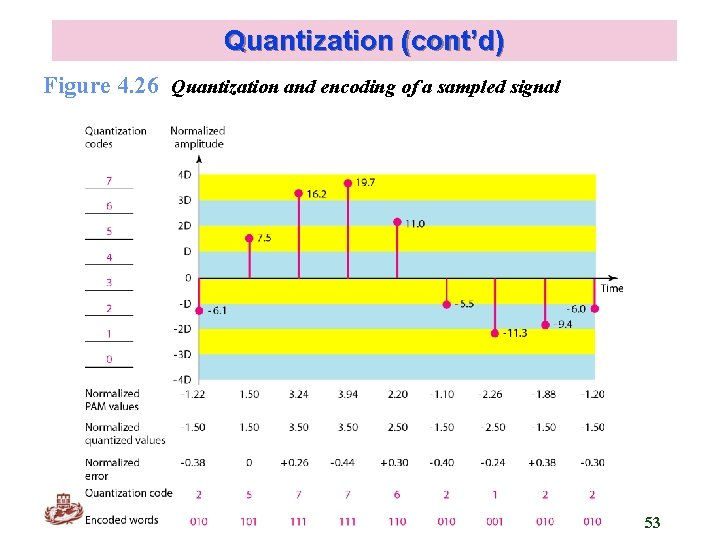Quantization (cont’d) Figure 4. 26 Quantization and encoding of a sampled signal Kyung Hee University 53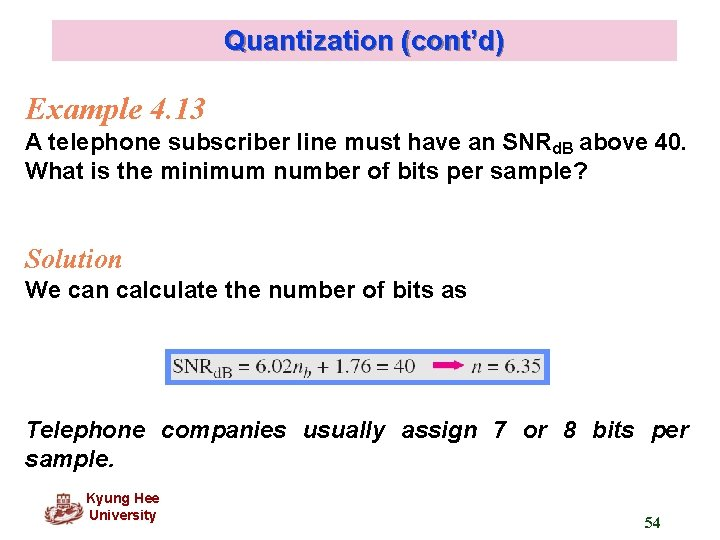Quantization (cont’d) Example 4. 13 A telephone subscriber line must have an SNRd. B above 40. What is the minimum number of bits per sample? Solution We can calculate the number of bits as Telephone companies usually assign 7 or 8 bits per sample. Kyung Hee University 54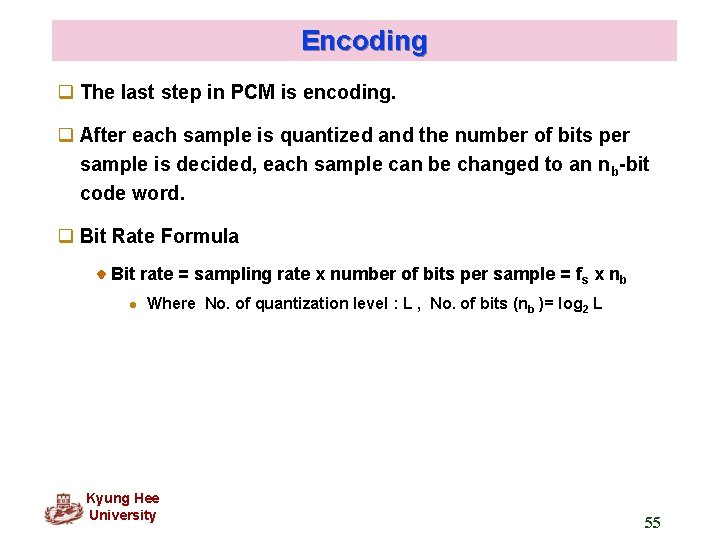Encoding q The last step in PCM is encoding. q After each sample is quantized and the number of bits per sample is decided, each sample can be changed to an nb-bit code word. q Bit Rate Formula Bit rate = sampling rate x number of bits per sample = fs x nb l Where No. of quantization level : L , No. of bits (n b )= log 2 L Kyung Hee University 55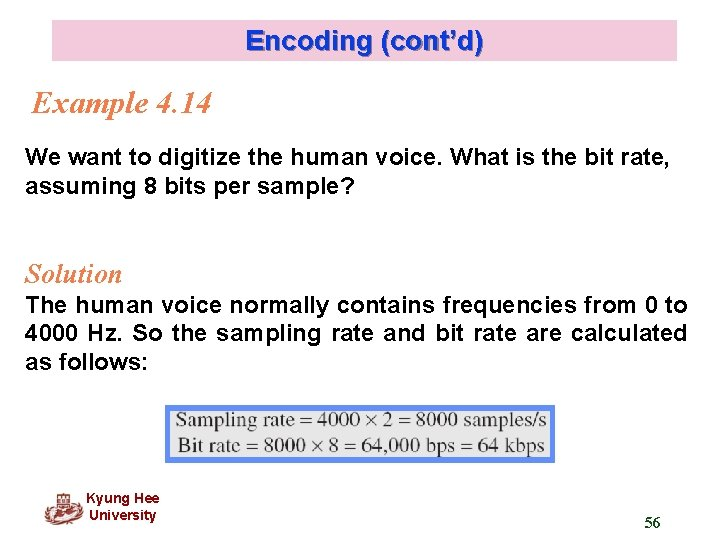Encoding (cont’d) Example 4. 14 We want to digitize the human voice. What is the bit rate, assuming 8 bits per sample? Solution The human voice normally contains frequencies from 0 to 4000 Hz. So the sampling rate and bit rate are calculated as follows: Kyung Hee University 56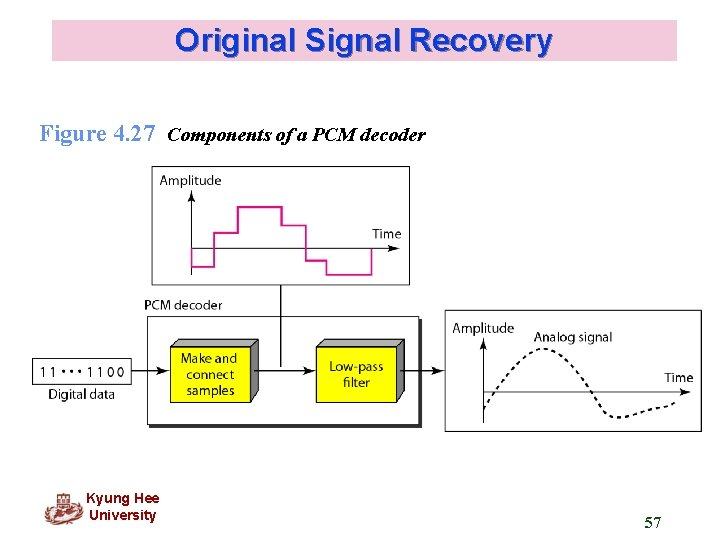Original Signal Recovery Figure 4. 27 Components of a PCM decoder Kyung Hee University 57PCM Bandwidth q If we digitized the signal, what is the new bandwidth of the channel ? Bmin = c x N x 1/r = c x nb x fs x 1/r = c x nb x 2 Banalog x 1/r q The minimum bandwidth (1/r = 1 for NRZ or bipolar, c= 1/2) Bmin = nb x Banalog q The maximum data rate of a Channel Nmax = 2 x B x log 2 L (bps) q The minimum bandwidth Bmin = N / (2 x log 2 L) (Hz) Kyung Hee University 58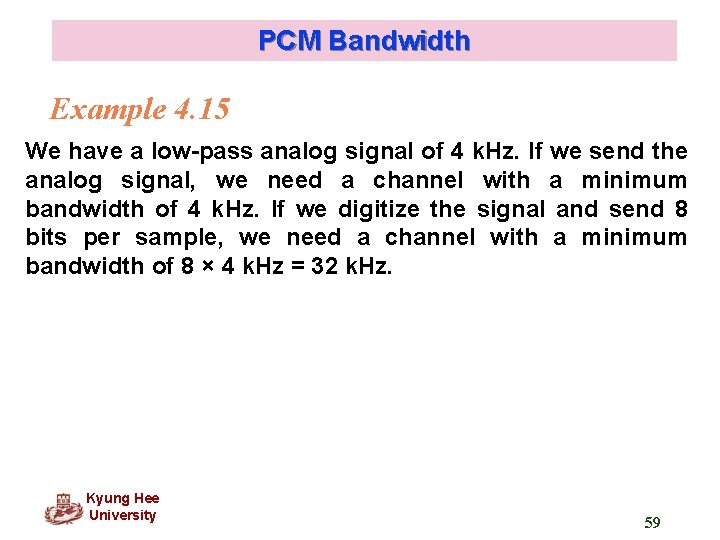PCM Bandwidth Example 4. 15 We have a low-pass analog signal of 4 k. Hz. If we send the analog signal, we need a channel with a minimum bandwidth of 4 k. Hz. If we digitize the signal and send 8 bits per sample, we need a channel with a minimum bandwidth of 8 × 4 k. Hz = 32 k. Hz. Kyung Hee University 59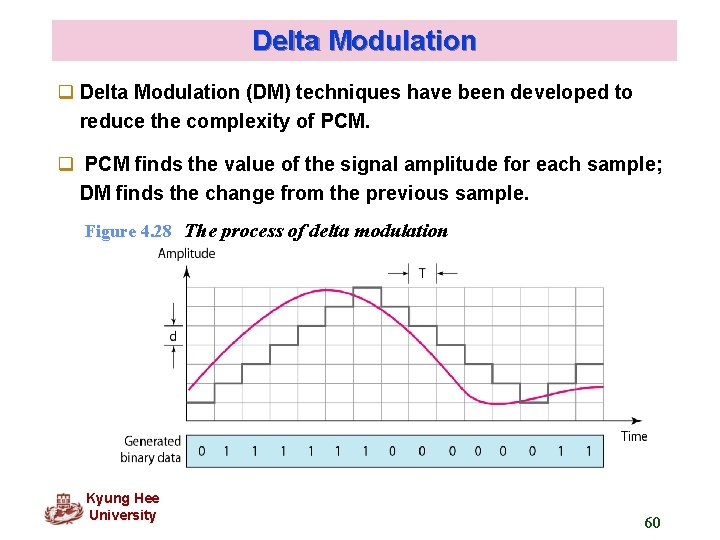Delta Modulation q Delta Modulation (DM) techniques have been developed to reduce the complexity of PCM. q PCM finds the value of the signal amplitude for each sample; DM finds the change from the previous sample. Figure 4. 28 The process of delta modulation Kyung Hee University 60Delta Modulation (cont’d) Figure 4. 29 Delta modulation components Kyung Hee University 61Delta Modulation (cont’d) Figure 4. 30 Delta demodulation components Kyung Hee University 624. 3 TRANSMISSION MODES The transmission of binary data across a link can be accomplished in either parallel or serial mode. In parallel mode, multiple bits are sent with each clock tick. In serial mode, 1 bit is sent with each clock tick. While there is only one way to send parallel data, there are three subclasses of serial transmission: asynchronous, and isochronous. Topics discussed in this section: Parallel Transmission Serial Transmission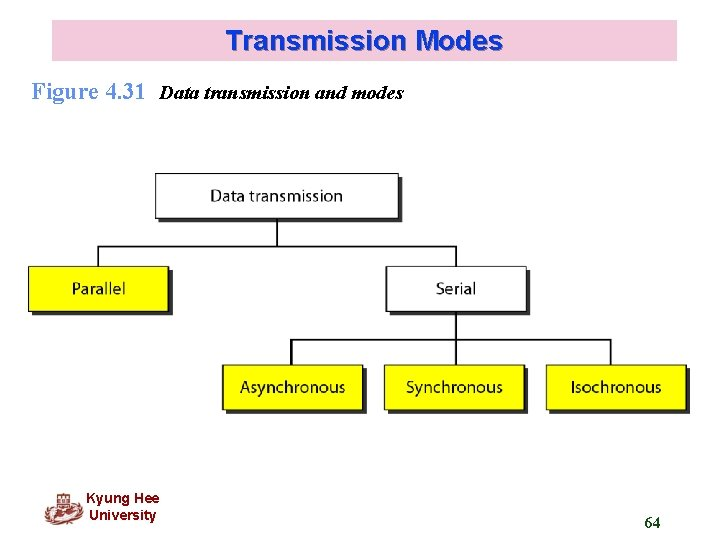Transmission Modes Figure 4. 31 Data transmission and modes Kyung Hee University 64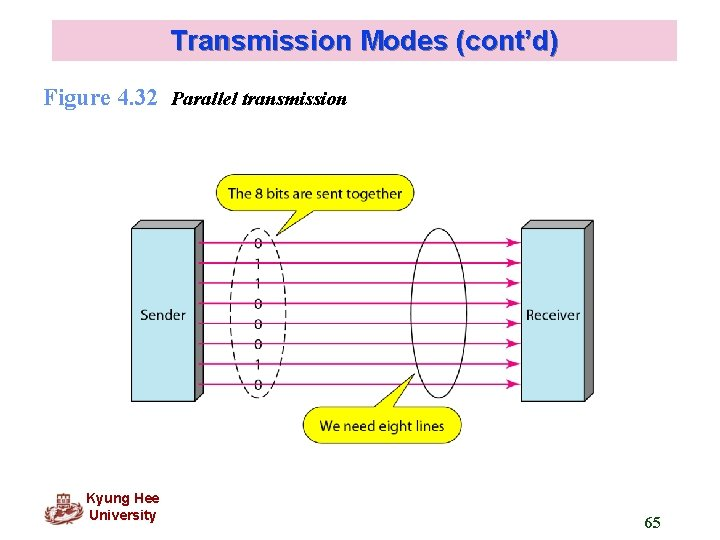Transmission Modes (cont’d) Figure 4. 32 Parallel transmission Kyung Hee University 65Transmission Modes (cont’d) Figure 4. 33 Serial transmission Kyung Hee University 66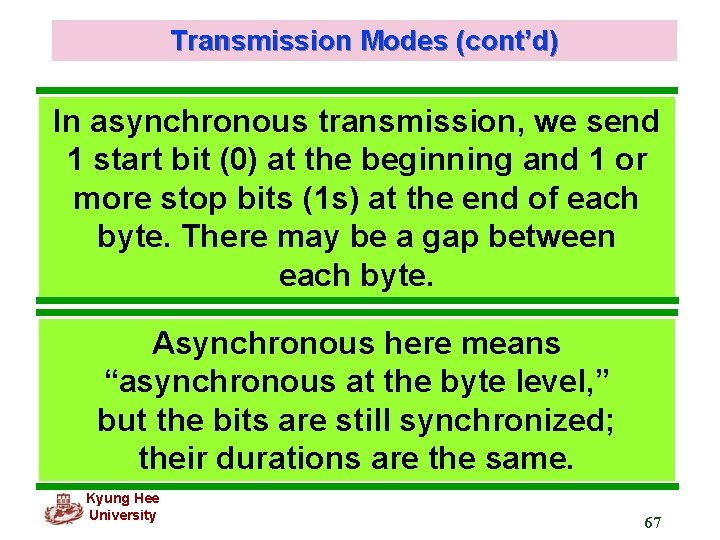Transmission Modes (cont’d) In asynchronous transmission, we send 1 start bit (0) at the beginning and 1 or more stop bits (1 s) at the end of each byte. There may be a gap between each byte. Asynchronous here means “asynchronous at the byte level, ” but the bits are still synchronized; their durations are the same. Kyung Hee University 67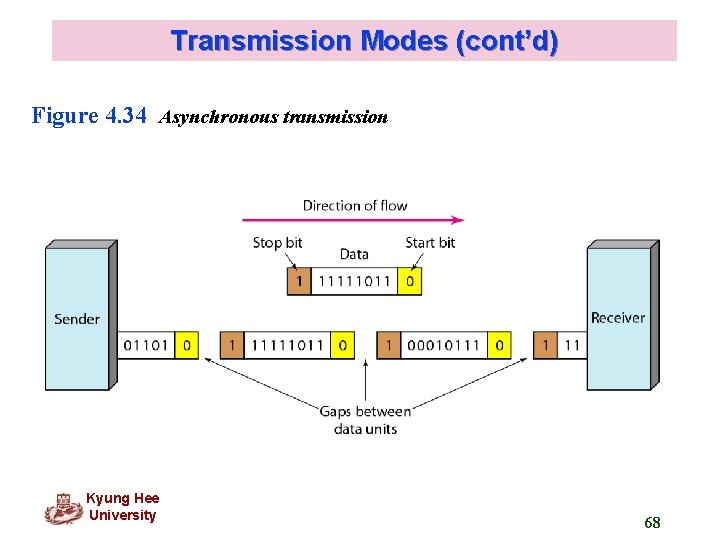Transmission Modes (cont’d) Figure 4. 34 Asynchronous transmission Kyung Hee University 68Transmission Modes (cont’d) In synchronous transmission, we send bits one after another without start or stop bits or gaps. It is the responsibility of the receiver to group the bits. Kyung Hee University 69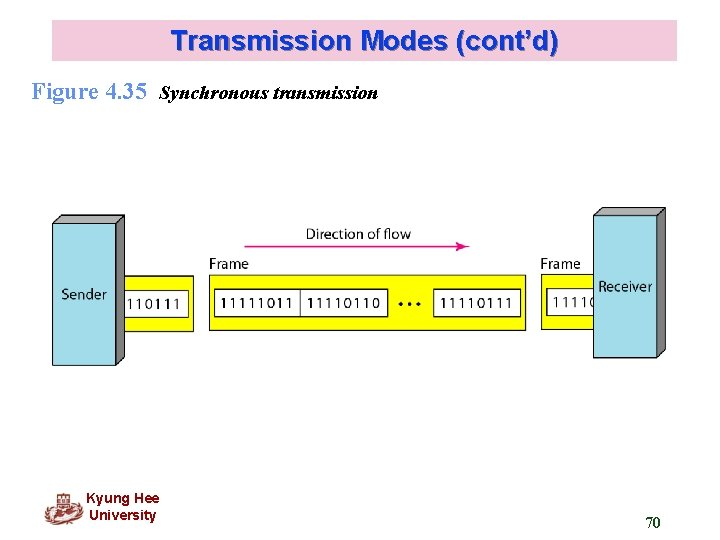Transmission Modes (cont’d) Figure 4. 35 Synchronous transmission Kyung Hee University 70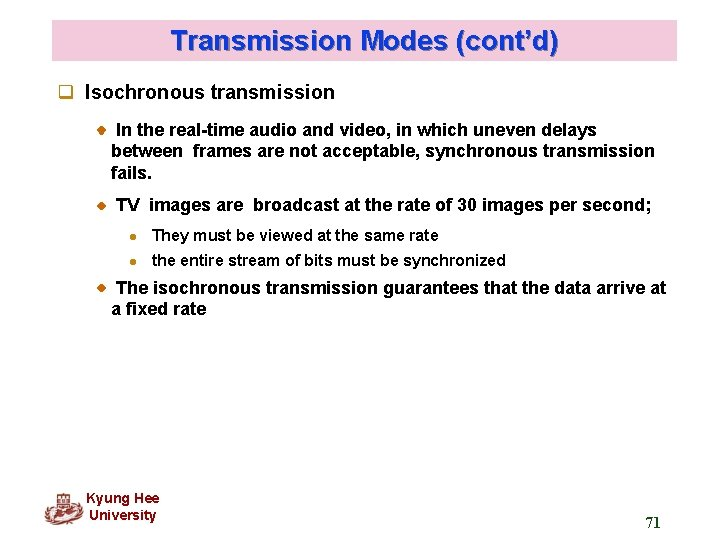Transmission Modes (cont’d) q Isochronous transmission In the real-time audio and video, in which uneven delays between frames are not acceptable, synchronous transmission fails. TV images are broadcast at the rate of 30 images per second; l They must be viewed at the same rate l the entire stream of bits must be synchronized The isochronous transmission guarantees that the data arrive at a fixed rate Kyung Hee University 71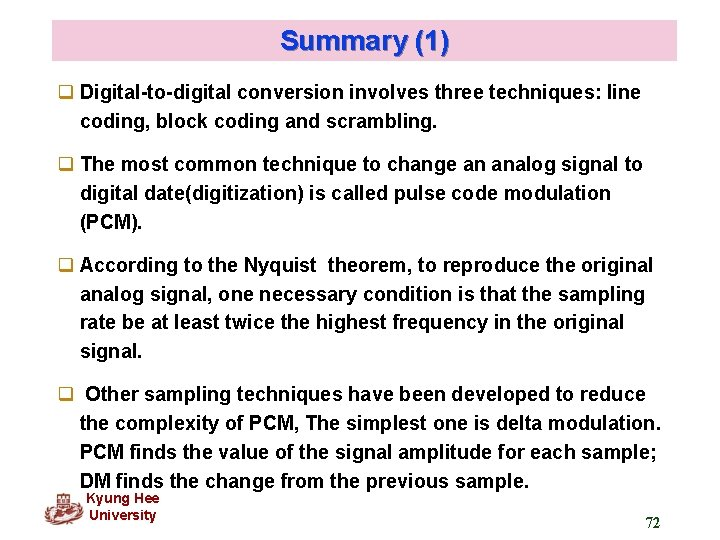Summary (1) q Digital-to-digital conversion involves three techniques: line coding, block coding and scrambling. q The most common technique to change an analog signal to digital date(digitization) is called pulse code modulation (PCM). q According to the Nyquist theorem, to reproduce the original analog signal, one necessary condition is that the sampling rate be at least twice the highest frequency in the original signal. q Other sampling techniques have been developed to reduce the complexity of PCM, The simplest one is delta modulation. PCM finds the value of the signal amplitude for each sample; DM finds the change from the previous sample. Kyung Hee University 72Summary(2) q There are three subclasses of serial transmission: asynchronous, and isochronous Kyung Hee University 73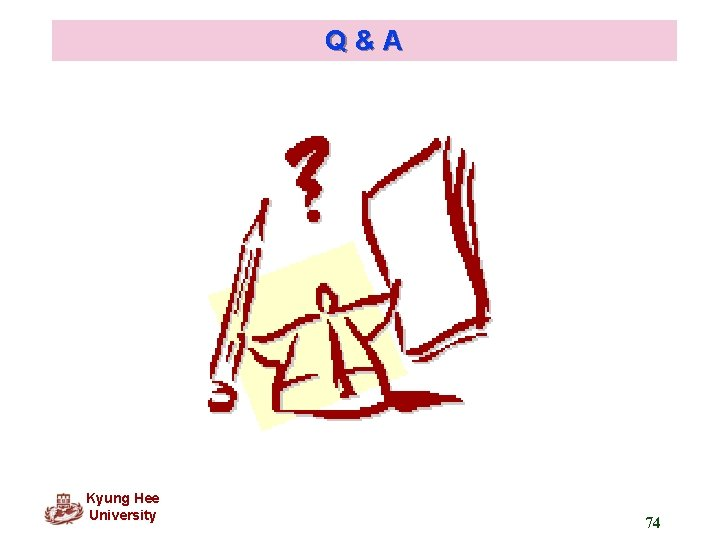Q&A Kyung Hee University 74